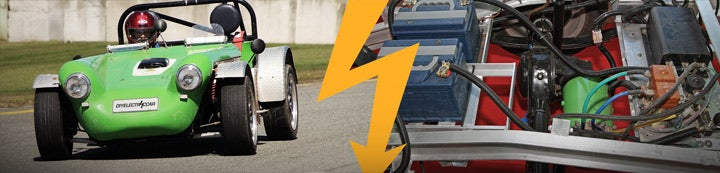1 - 2 of 2 Posts

#### iugmoh

·
##### Registered
Joined
·
2 Posts
Discussion Starter
hi all,
I need your help, experience, references, or hints on how to estimate the parameters for any DC motor , like these parameters
----------------------------------------------------
These are the motor parameters that are needed:
Motor torque constant KT (lb-in/amp)
Motor resistance Ra (ohms)
Motor inductance La (Henries)
Motor inertia Jm (lb-in-sec^2)
-----------------------------------------------------
because sometimes we find a dc motor from any source and need to make any control project but you need its parameters to work, so if one have any information will be thanks

#### major

·
##### Registered
Joined
·
7,837 Posts
hi all,
I need your help, experience, references, or hints on how to estimate the parameters for any DC motor , like these parameters
----------------------------------------------------
These are the motor parameters that are needed:
Motor torque constant KT (lb-in/amp)
Motor resistance Ra (ohms)
Motor inductance La (Henries)
Motor inertia Jm (lb-in-sec^2)
-----------------------------------------------------
Hi iugmoh,

The motor torque and voltage constants are equal in metric units.

K = (p*N)/(2*pi*a)

Where:
p = # of poles
N = # of conductors
2 = 2
pi = 3.14
a = # of current paths

Ra can be calculated from the wire size, turns and geometey, but easier and more accurate to measure.

La is even harder to calculate and even difficult to measure due to saturation effects.

The rotor and load inertias can be calculated using the size, shape and mass. See a physics book for details.

Regards,

major

1 - 2 of 2 Posts